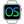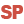• WLanguage properties that can be used with the Point type
• Assigning values to a Point variable
• Assigning values to an array of Points
• Comparison and serialization
• Notes
WINDEVWEBDEVWINDEV MobileOthersPoint (Type of variable)
In french: Point
Point is a structured type used to represent a position (X, Y) in 2 dimensions.
Example
MyPoint is Point
MyPoint.X = 280
MyPoint.Y = 54
Syntax

Declaring and initializing a point

<Point name> is Point
<Point name>:
Name of the Point variable to declare.
Remarks

WLanguage properties that can be used with the Point type

The initial value of a point is set to (0,0).
The following properties can be used to handle a Point variable:
Property name Effect X X-coordinate of the point. This coordinate corresponds to a signed 4-byte integer. Y Y-coordinate of the point. This coordinate corresponds to a signed 4-byte integer.

Assigning values to a Point variable

The following values can be assigned to a Point variable:
• another point.
For example:
MyPoint is Point
MyPoint.X = 280
MyPoint.Y = 54
MyPoint1 is Point = MyPoint
• an array of two values that can be converted to integers.
For example:
MyPoint is Point
MyPoint = [280, 54]

Assigning values to an array of Points

The following values can be assigned to an array of Points:
• an array of values containing points.
For example:
ArrayOfPoints is array of Point
ArrayOfPoints = [MyPoint1, MyPoint2]
• an array of two values that can be converted to integers.
For example:
ArrayOfPoints is array of Point
ArrayOfPoints = [[1,2],[3,4]]

Comparison and serialization

• Comparison: The = and <> comparison operators are available.
• Serialization: The binary, XML and JSON serializations are available.
Caution: In an XML serialization, xmlAttribute cannot be used on a structure or class member of type Point.

Notes

• A Point variable can be stored in a variant.
• A Point variable cannot hold Null values:
• The "PointVariable = NULL" condition always returns False.
• The "PointVariable <> NULL" condition always returns True.
• You cannot reference a Point variable.
The "PointVariable2 <- PointVariable1" syntax triggers an error.
• You cannot force the copy of a Point variable.
The "PointVariable2 <= PointVariable1" syntax triggers an error.
• You can add and subtract two Point variables.
• Point variables can be passed to the system APIs.
• Point variables are used to define the points of Polygon variables.
Minimum version required
• Version 21